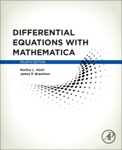## Mathematical Sciences Faculty Bookshelf#### Title

Differential Equations with Mathematica

#### Files

Download Full Text

#### Description

Georgia Southern faculty members Martha L. Abell and James P. Braselton co-authored Differential Equations with Mathematica, 4th Edition.

Differential Equations with Mathematica, Fourth Edition is a supplementing reference which uses the fundamental concepts of the popular platform to solve (analytically, numerically, and/or graphically) differential equations of interest to students, instructors, and scientists.

Mathematica’s diversity makes it particularly well suited to performing calculations encountered when solving many ordinary and partial differential equations. In some cases, Mathematica’s built-in functions can immediately solve a differential equation by providing an explicit, implicit, or numerical solution. In other cases, mathematica can be used to perform the calculations encountered when solving a differential equation.

Because one goal of elementary differential equations courses is to introduce students to basic methods and algorithms so that they gain proficiency in them, nearly every topic covered this book introduces basic commands, also including typical examples of their application. A study of differential equations relies on concepts from calculus and linear algebra, so this text also includes discussions of relevant commands useful in those areas. In many cases, seeing a solution graphically is most meaningful, so the book relies heavily on Mathematica’s outstanding graphics capabilities.

• Demonstrates how to take advantage of the advanced features of Mathematica 10
• Introduces the fundamental theory of ordinary and partial differential equations using Mathematica to solve typical problems of interest to students, instructors, scientists, and practitioners in many fields
• Showcases practical applications and case studies drawn from biology, physics, and engineering

9-13-2016

Academic Press

#### ISBN for this edition (13-digit)

9780128047767COinS